Latest Banking jobs   »

# Quantitative Aptitude Quiz For RBI Grade B Phase 1 2023 -26th February

Q1. Ratio of numerical value of rate of interest and time period is 4 : 1. Man invested Rs. 2400 and gets Rs. 864 as simple interest. Find the value of X, if man invested Rs. (2400 + X) at same rate of interest on C.I. for two years and get Rs. 814.08 as interest?
(a) 600 Rs.
(b) 800 Rs.
(c) 400 Rs.
(d) 200 Rs.
(e) 540 Rs.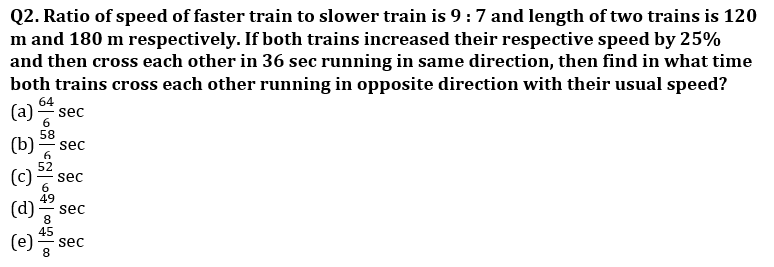Q3. A and B entered into a business with the capital of Rs. (X + 1200) and Rs (X+1500) respectively. After eight months from starting A withdraw half of his investment and B doubled his investment. If at the end of the year A got Rs. 4250 as profit share out of total profit of Rs. 11250, then find three times of initial investment of B ?
(a) Rs 32,400
(b) Rs 30,750
(c) Rs 30,500
(d) Rs 31,500
(e) Rs 31,770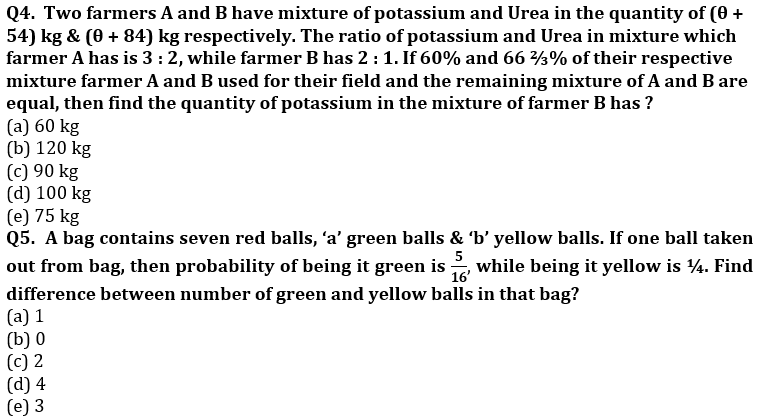Q6. Mohan mixed two types of wheats costing Rs. 80 per kg and Rs. 120 per kg in the ratio of 1 : 3 and sold this mixture at the price of Rs. 143 per kg. Find the profit percentage of Mohan?
(a) 25%
(b) 30%
(c) 35%
(d) 40%
(e) 20%
Q7. Gurdeep chhabra joined ‘Adda 247’ with the work experience of 26 years due to which average work experience of all employees of ‘Adda 247’ was increased by one year. If initial average work experience of all employees of ‘Adda 247’ was five years, then find the new number of employees in ‘Adda 247’?
(a) 23
(b) 19
(c) 25
(d) 21
(e) 27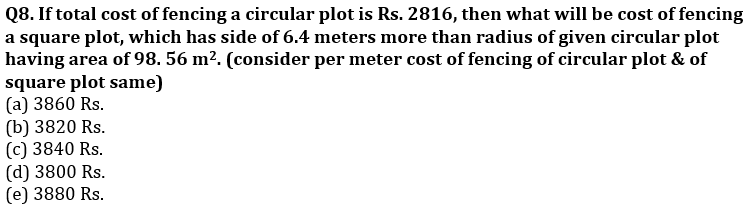Q9. Ram and Shyam invest in the ratio of 3 : 4 in a partnership business and Ram got Rs. 2700 as profit share out of total profit of Rs. 5100, then find the ratio of period of investment of Shyam to that of Ram?
(a) 3 : 4
(b) 3 : 5
(c) 2 : 3
(d) 1 : 2
(e) 3 : 2
Q10. A wooden seller has some number of tables which are 25% more than number of chairs. 60% of tables and 50% of chairs are sold, each table is at Rs. 250 and each chair is at Rs. 240. If number of sold tables are 30 more than that of sold chair, then find difference between total selling price of sold tables and sold chairs (in Rs.)?
(a) 8200
(b) 8100
(c) 9100
(d) 8400
(e) 8500

Direction (11-15): In each of the questions below, you have to find the value of question mark. You are not supposed to find the exact value.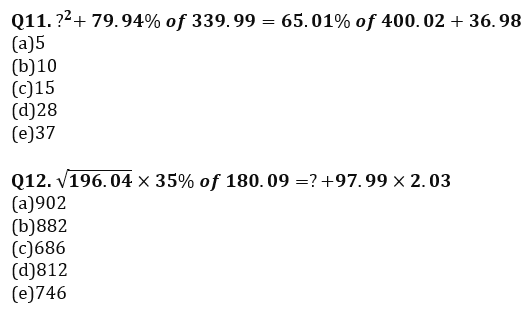Q13. 42% of 799.89-29.99% of 650.04+?=439.99÷2
(a)55
(b)97
(c)108
(d)23
(e)79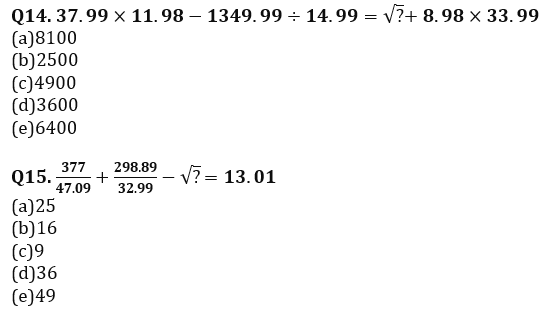Solutions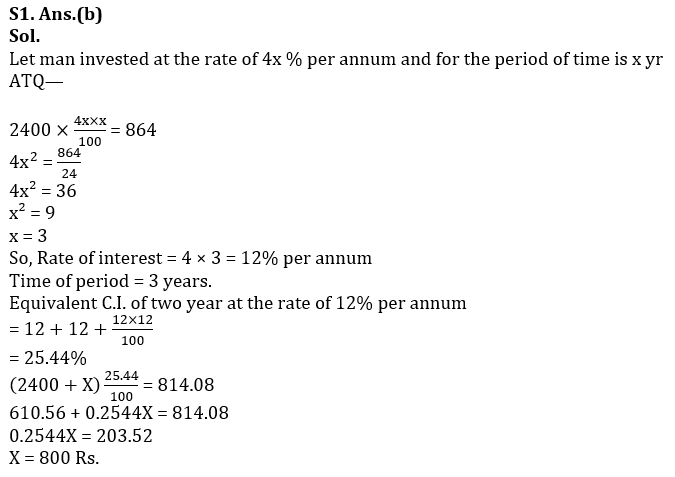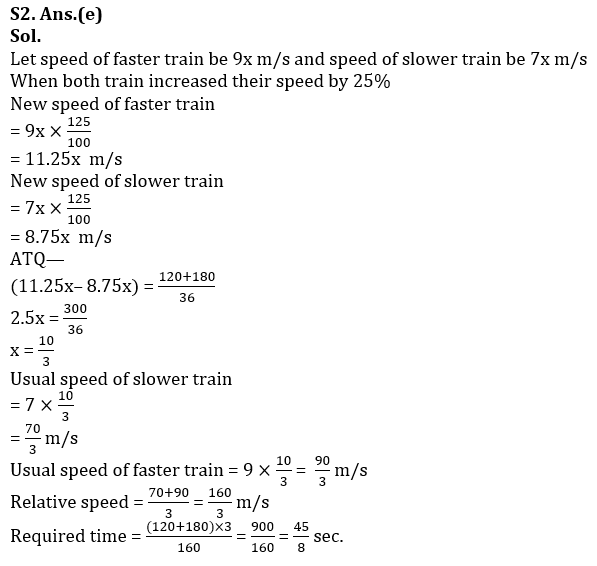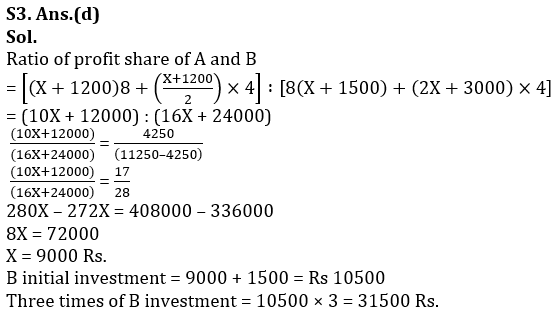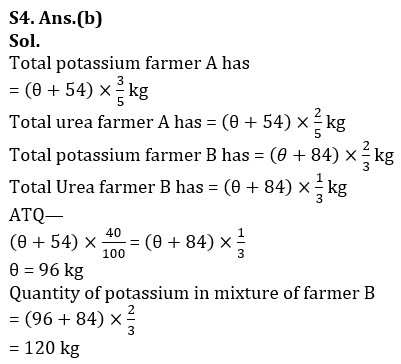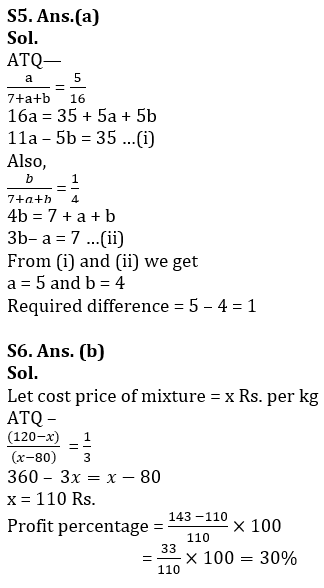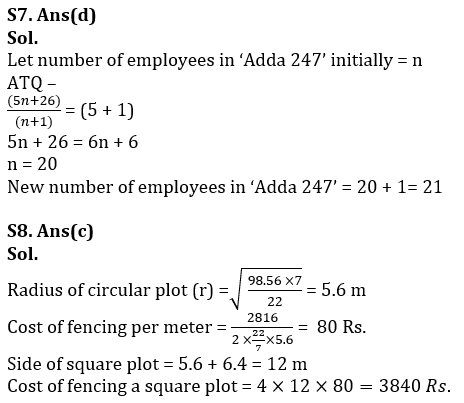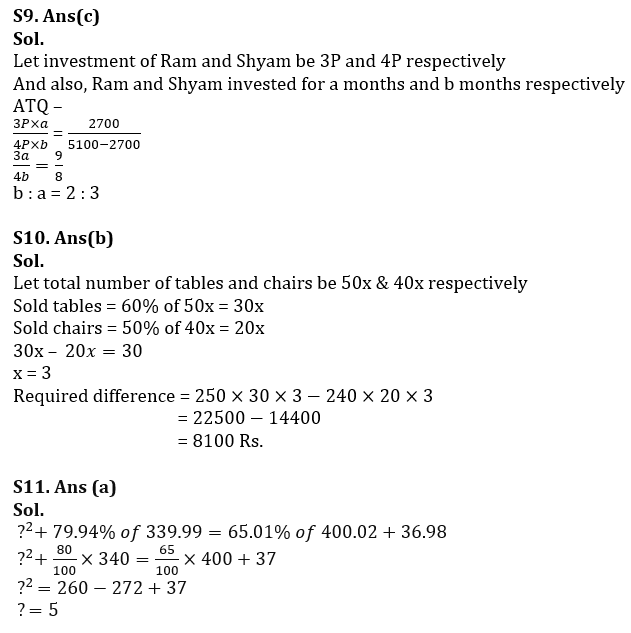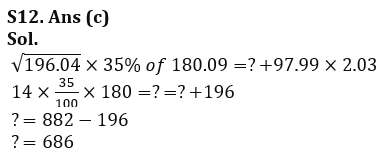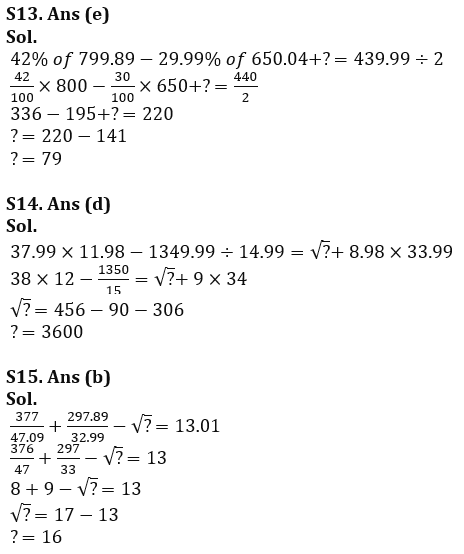## FAQs

### How many sections are there in the RBI Grade B Phase 1 Exam?

There are 4 sections in the RBI Grade B Phase 1 Exam i.e. English Language, General Awareness, Quantitative Aptitude & Reasoning.

#### Congratulations!Union Budget 2023-24: Free PDF